# Power Amplifier Characterization with DPD for Reduced Signal Distortion

This example provides a methodology for characterizing a nonlinear RF Blockset™ power amplifier (PA) with memory and an adaptive DPD feedback system to reduce the output signal distortion of an RF transmitter. The initial step in the procedure determines the `Coefficient matrix` required by the RF Power Amplifier block when selecting the Volterra memory polynomial model with cross-terms [ 1 ]. This step uses actual measured data for the PA. After deriving the set of coefficients for the PA model, a system-level simulation is performed. This system includes an adaptive DPD algorithm that can be enabled during simulation using the toggle switch and demonstrates how the linearity of the RF transmitter system output signal improves after enabling DPD correction.

### Read and Visualize Measured PA Input/Output Data

First, the input and output complex measured I/Q signals of the PA are loaded. A commercially available power amplifier has been characterized in the lab using a standard-compliant LTE signal with sample rate equal to 15.36 MHz.

```load('simrfV2_powamp_dpd_data.mat') DataRate = 15.36e6; Tstep = 1/DataRate; ```

The physical amplifier behavior is now visualized. The following figure plots the absolute value of the input and output signals as a function of time.

```numDataPts = length(inDataPA); plot((1:numDataPts)*Tstep, abs(inDataPA), ... (1:numDataPts)*Tstep, abs(outDataPA)) legend('Abs(In)','Abs(Out)','Location','northeast') xlabel('Time (s)') xlim([0 1e-5]) ylabel('Voltage (V)') title('Absolute Values of Input and Output Voltage Signals') ```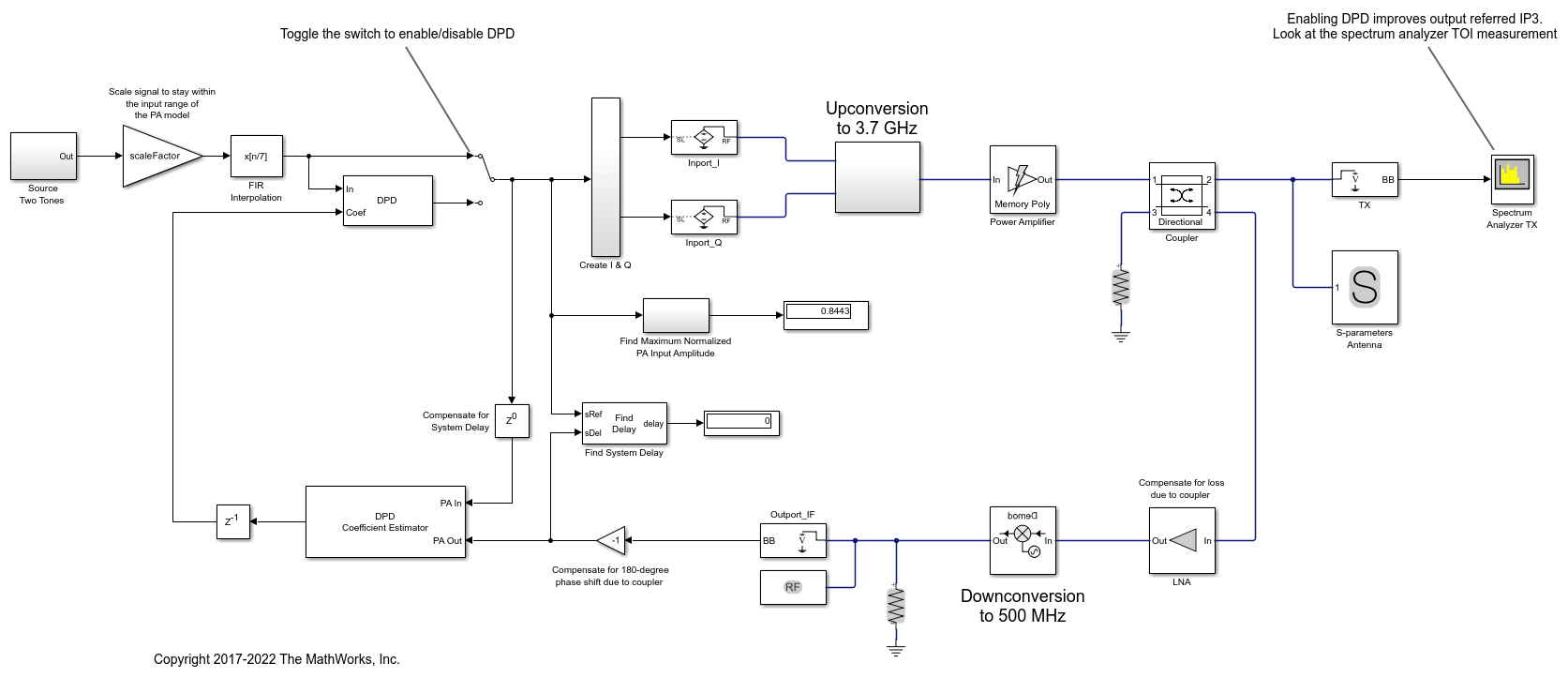The following figure plots the magnitude characteristic of the PA: the absolute value of the output gain versus input signals. In general, scattering around a straight line representing the gain results from memory effects (curve dispersion) and the bending of the data curve for large values of input signal results from the PA nonlinearity (deviation from straight horizontal line).

```TransferPA = abs(outDataPA./inDataPA); plot(abs(inDataPA),20*log10(TransferPA),'.') xlabel('Input Voltage Absolute Value(V)') ylabel('Magnitude Power Gain (dB)') title('Power Gain Transfer Function') ```### Determine PA Block Coefficient Matrix From Measured Input and Output Signals

Use the multipurpose helper function simrfV2_powamp_dpd_helper to determine the complex coefficients of a memory polynomial model for the amplifier characteristics. The memory length and degree of the model are empirically chosen here, but it is possible to automate this choice.

```memLen = 3; degLen = 7; ```

Only half of the data is used to compute the fitting coefficients, as the whole data set will be used to compute the relative error.

```halfDataPts = round(numDataPts/2); ```

The helper function supports the possibility to chose different memory models. As an example, to use the memory polynomial without cross terms:

```modType = 'memPoly'; ```

The helper function simrfV2_powamp_dpd_helper is editable for custom modifications, and to return the desired matrix.

```fitCoefMat = simrfV2_powamp_dpd_helper('coefficientFinder', ... inDataPA(1:halfDataPts),outDataPA(1:halfDataPts),memLen,degLen,modType); ```

To validate the fitting, use the helper function to compute an error estimate that consists the standard deviation of the predicted signal versus the measured signal.

```[errSig] = simrfV2_powamp_dpd_helper('errorMeasure', ... inDataPA, outDataPA, fitCoefMat, modType); disp(['Signal standard deviation = ' num2str(errSig) '%']) ```
```Signal standard deviation = 4.8083% ```

To visualize both the measured output signal and the fitted output signal, plot the time-domain output voltage.

```outDataPA_fit = simrfV2_powamp_dpd_helper('signalGenerator', ... inDataPA, fitCoefMat, modType); plot((1:numDataPts)*Tstep, abs(outDataPA), 'o-', ... (1:numDataPts)*Tstep, abs(outDataPA_fit), '.-') xlabel('Time (s)') ylabel('Voltage (V)') xlim([0 1e-5]) legend('Abs(Out)','Abs(OutFit)','Location','northeast') title('Absolute Values of Output and Fitted Output Signals') ```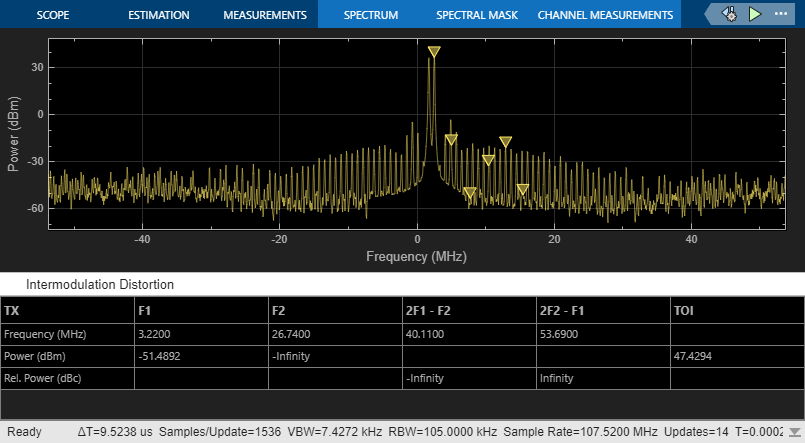We also plot the magnitude of the power transfer function:

```TransferPA_fit = abs(outDataPA_fit./inDataPA(:)); plot(abs(inDataPA), 20*log10(TransferPA), 'o', ... abs(inDataPA), 20*log10(TransferPA_fit), '.') xlabel('Input Voltage Absolute Value(V)') ylabel('Magnitude Power Gain (dB)') legend('Abs Gain','Abs Gain Fit','Location','northeast') title('Power Gain Transfer Function') ```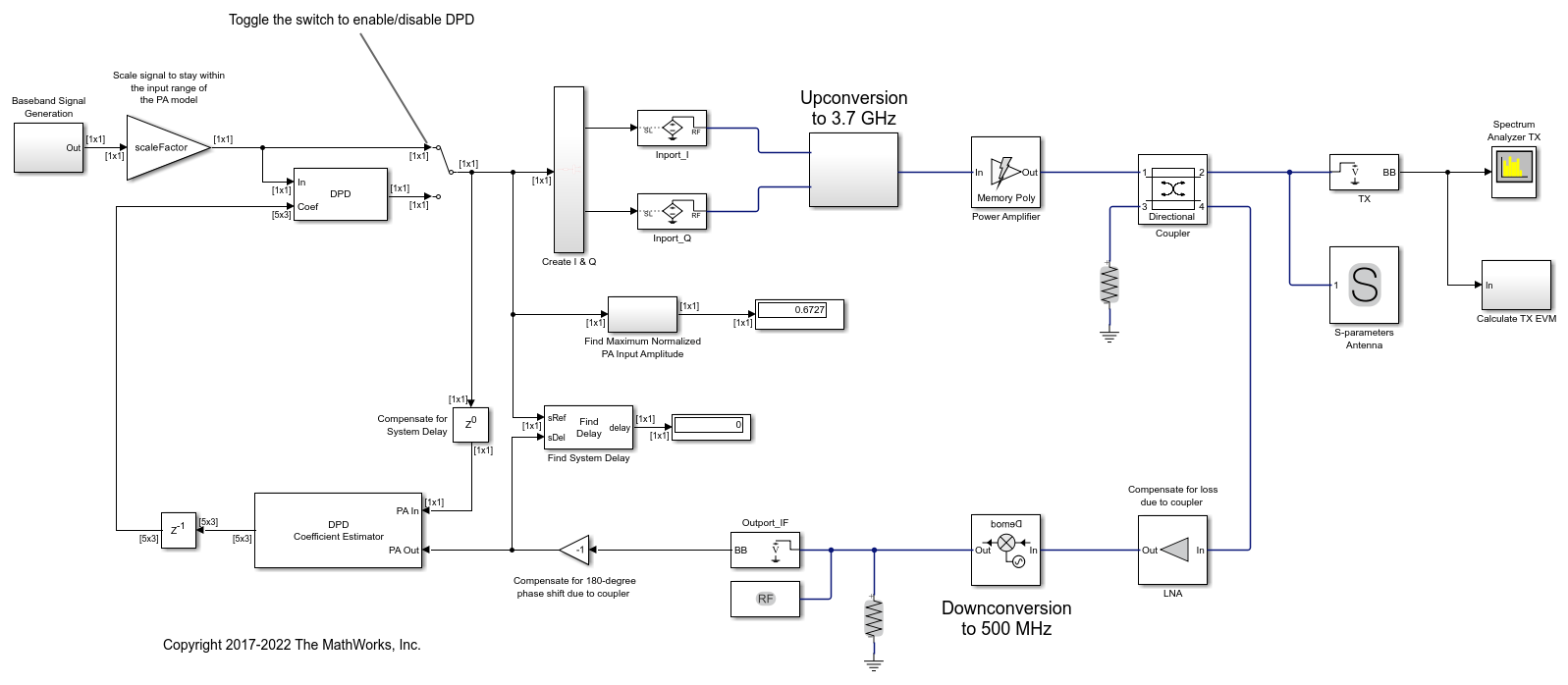### Improve Fitting with Memory Polynomial Model Including Cross Terms

We now determine the fitted coefficient matrix from the input and output characteristics by using a different approach that includes leading and lagging memory cross terms.

We use the same helper function, but we use a different model type.

```modType = 'ctMemPoly'; fitCoefMat = simrfV2_powamp_dpd_helper('coefficientFinder', ... inDataPA(1:halfDataPts),outDataPA(1:halfDataPts),memLen,degLen,modType); ```

To validate the fitting, we compute the error. Use the helper function to compute the standard deviation of the predicted signal versus the measured signal. The error is now lower thanks to the leading and lagging memory cross terms.

```[errSig] = simrfV2_powamp_dpd_helper('errorMeasure', ... inDataPA, outDataPA, fitCoefMat, modType); disp(['Signal standard deviation = ' num2str(errSig)]) ```
```Signal standard deviation = 2.7045 ```

To verify that the fitting has higher quality and to visualize the measured output signal and the fitted output signal, we plot the data.

```outDataPA_fit = simrfV2_powamp_dpd_helper('signalGenerator', ... inDataPA, fitCoefMat, modType); plot((1:numDataPts)*Tstep, abs(outDataPA), 'o-', ... (1:numDataPts)*Tstep, abs(outDataPA_fit), '.-') xlabel('Time (s)') ylabel('Voltage (V)') xlim([0 1e-5]) legend('Abs(Out)','Abs(OutFit)','Location','northeast') title('Absolute Values of Output and Fitted Output Signals') ``````TransferPA_fit = abs(outDataPA_fit./inDataPA(:)); plot(abs(inDataPA), 20*log10(TransferPA), 'o', ... abs(inDataPA), 20*log10(TransferPA_fit), '.') xlabel('Input Voltage Absolute Value(V)') ylabel('Magnitude Power Gain (dB)') legend('Abs Gain','Abs Gain Fit','Location','northeast') title('Power Gain Transfer Function') ```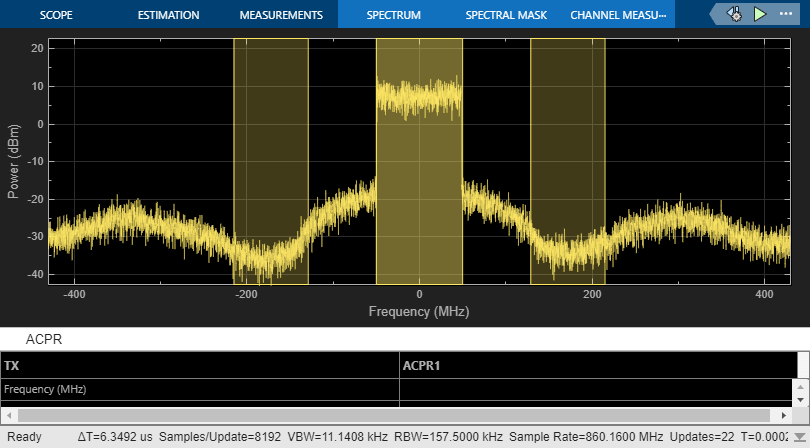At last, we save the coefficient matrix of the PA model that we will import and use in RF Blockset for simulation at the system-level.

```save('PAcoefficients.mat','fitCoefMat') ```

### System Level Model Including PA and DPD with Two Sinusoidal Test Signals

Open the Simulink RF Blockset model including the PA model and the adaptive DPD algorithm [ 2 ]:

System-level model PA+DPD

The model includes a two-tone signal generator that is used for testing the output-referred third-order intercept point of the system. The model includes upconversion to RF frequency using an I-Q modulator, the PA model with the coefficients that we fitted, a coupler to sniff the output of the PA, and an S-parameter block representing the antenna loading effect. The receiver chain performs downconversion to low intermediate frequency. Notice that the simulation bandwidth of this system is 50 MHz.

The model can be simulated without the DPD when the toggle switch is in the up position.

```model = 'simrfV2_powamp_dpd'; open_system(model) sim(model) ```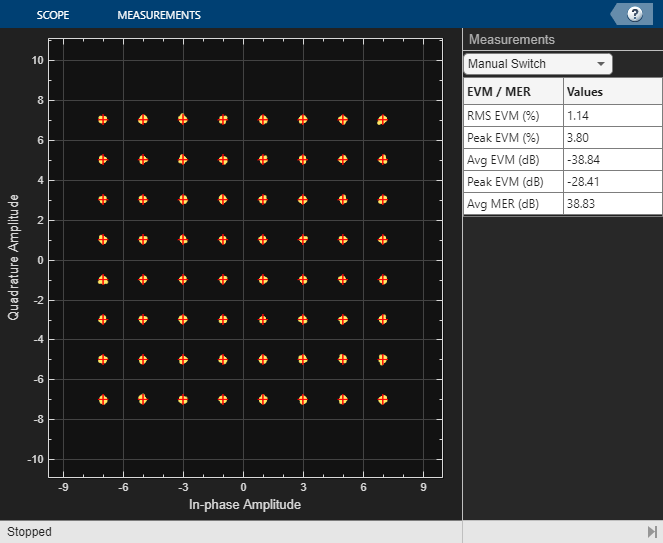The manual switch is toggled to enable the DPD algorithm. When toggled, the TOI (third-order intercept point) improves from 36 dBm to approximately 48 dBm within 0.5 ms of simulation time. Inspect the distortion measurement in the Spectrum Analyzer to validate these results and see how the power of the harmonics is reduced thanks to the DPD linearization.

```set_param([model '/Manual Switch'], 'action', '1') sim(model) ```By changing the degree and the memory depth defined in the DPD Coefficient Estimator block, you can find the most suitable tradeoff between performance and implementation cost.

To estimate the DPD coefficients correctly, the input signals to the DPD Coefficient Estimator block, PA In and PA Out, must be aligned in the time domain. This is verified by the Find Delay block which shows that the delay introduced by the RF system is 0. The estimator's desired amplitude gain is important to make sure that the total linear gain of the system does not change when the DPD is active. For this particular model, the gain has been estimated using separate techniques [ 2 ].

```close_system(model,0) close all; clear ```

### System Level Model Including PA and DPD with 16-QAM Waveform

Open the Simulink RF Blockset model including the PA model and the adaptive DPD algorithm excited with a 16-QAM modulated waveform.

System-level model PA+DPD with 16-QAM

Without DPD linearization, the system has a Modulation Error Ratio of 15 dB, as it can be seen from the constellation plot measurement.

```model = 'simrfV2_powamp_dpd_comms'; open_system(model) sim(model) ```The manual switch is toggled to enable the DPD algorithm. When toggled, the average MER improves from 15 dB to approximately 28 dB within 0.5 ms of simulation time.

```set_param([model '/Manual Switch'], 'action', '1') sim(model) ``````close_system(model,0) close all; clear ```

### Selected Bibliography

1. Morgan, Dennis R., Zhengxiang Ma, Jaehyeong Kim, Michael G. Zierdt, and John Pastalan. "A Generalized Memory Polynomial Model for Digital Predistortion of Power Amplifiers." IEEE® Transactions on Signal Processing. Vol. 54, No. 10, October 2006, pp. 3852–3860.

2. Gan, Li, and Emad Abd-Elrady. "Digital Predistortion of Memory Polynomial Systems Using Direct and Indirect Learning Architectures." In Proceedings of the Eleventh IASTED International Conference on Signal and Image Processing (SIP) (F. Cruz-Roldán and N. B. Smith, eds.), No. 654-802. Calgary, AB: ACTA Press, 2009.